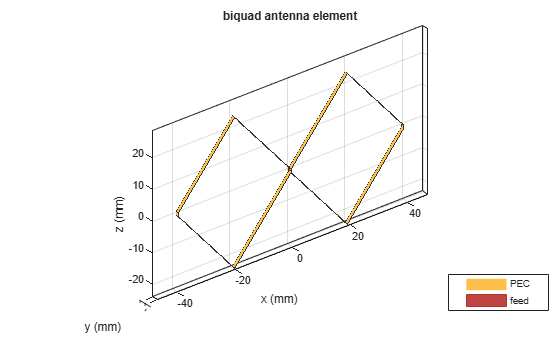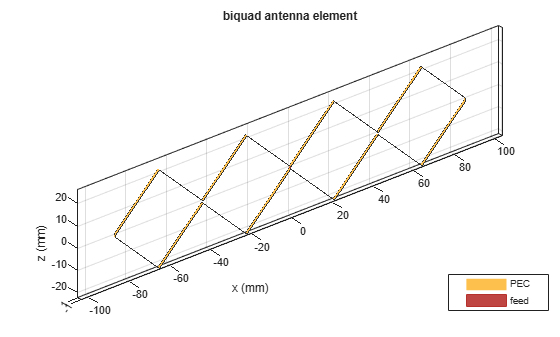## Description

The `biquad` antenna is center fed and symmetric about its origin. The default length is chosen for an operating frequency of 2.8 GHz.

The width of the strip is related to the diameter an equivalent cylinder:

`$w=2d=4r$`

, where:

• d is the diameter of equivalent cylindrical dipole.

• r is the radius of equivalent cylindrical dipole.

For a given cylinder radius, use the `cylinder2strip` utility function to calculate the equivalent width. The default strip dipole is center-fed. The feed point coincides with the origin. The origin is located on the Y-Z plane.## Creation

### Syntax

``bq = biquad``
``bq = biquad(Name,Value)``

### Description

````bq = biquad` creates a biquad antenna.```

example

``` `bq = biquad(Name,Value)` creates a biquad antenna with additional properties specified by one or more name-value pair arguments. `Name` is the property name and `Value` is the corresponding value. You can specify several name-value pair arguments in any order as `Name1`, `Value1`, `...`, `NameN`, `ValueN`. Properties not specified retain their default values.```

## Properties

expand all

Number of loops for the biquad, specified as a scalar integer. Setting this property to 4 supports a double biquad antenna.

Example: `'NumLoops'`,`4`

Data Types: `double`

Length of two arms, specified as a scalar in meters. The default length is chosen for an operating frequency of 2.8 GHz.

Example: `'ArmLength'`,`0.0206`

Data Types: `double`

Biquad arm width, specified as a scalar in meters.

Example: `'Width'`,`0.006`

Data Types: `double`

Angle formed by biquad arms to the X-Y plane, specified a scalar in meters.

Example: `'ArmElevation'`, `50`

Data Types: `double`

Lumped elements added to the antenna feed, specified a lumped element object handle. For more information, see `lumpedElement`.

Example: `'Load'`, lumpedelement. `lumpedelement` is the object handle for the load created using `lumpedElement`.

Example: ```bq.Load = lumpedElement('Impedance',75)```

Tilt angle of the antenna, specified as a scalar or vector with each element unit in degrees. For more information, see Rotate Antennas and Arrays.

Example: `'Tilt',90`

Example: `ant.Tilt = 90`

Example: `'Tilt',[90 90]`,`'TiltAxis',[0 1 0;0 1 1]` tilts the antenna at 90 degrees about the two axes defined by the vectors.

Note

The `wireStack` antenna object only accepts the dot method to change its properties.

Data Types: `double`

Tilt axis of the antenna, specified as:

• Three-element vector of Cartesian coordinates in meters. In this case, each coordinate in the vector starts at the origin and lies along the specified points on the X-, Y-, and Z-axes.

• Two points in space, each specified as three-element vectors of Cartesian coordinates. In this case, the antenna rotates around the line joining the two points in space.

• A string input describing simple rotations around one of the principal axes, 'X', 'Y', or 'Z'.

Example: `'TiltAxis',[0 1 0]`

Example: `'TiltAxis',[0 0 0;0 1 0]`

Example: `ant.TiltAxis = 'Z'`

Note

The `wireStack` antenna object only accepts the dot method to change its properties.

Data Types: `double`

## Object Functions

 `show` Display antenna or array structure; display shape as filled patch `info` Display information about antenna or array `axialRatio` Axial ratio of antenna `beamwidth` Beamwidth of antenna `charge` Charge distribution on metal or dielectric antenna or array surface `current` Current distribution on metal or dielectric antenna or array surface `design` Design prototype antenna or arrays for resonance at specified frequency `EHfields` Electric and magnetic fields of antennas; Embedded electric and magnetic fields of antenna element in arrays `impedance` Input impedance of antenna; scan impedance of array `mesh` Mesh properties of metal or dielectric antenna or array structure `meshconfig` Change mesh mode of antenna structure `optimize` Optimize antenna or array using SADEA optimizer `pattern` Radiation pattern and phase of antenna or array; Embedded pattern of antenna element in array `patternAzimuth` Azimuth pattern of antenna or array `patternElevation` Elevation pattern of antenna or array `returnLoss` Return loss of antenna; scan return loss of array `sparameters` S-parameter object `vswr` Voltage standing wave ratio of antenna

## Examples

collapse all

Create a biquad antenna with arm angles at 50 degrees and view it.

```bq = biquad('ArmElevation',50); show(bq)```Calculate the impedance of a biquad antenna over a frequency span 2.5GHz-3GHz.

```bq = biquad('ArmElevation',50); impedance(bq,linspace(2.5e9,3e9,51));```Create and view a double biquad antenna using default property values.

`ant = biquad('NumLoops',4)`
```ant = biquad with properties: NumLoops: 4 ArmLength: 0.0305 ArmElevation: 45 Width: 1.0000e-03 Tilt: 0 TiltAxis: [1 0 0] Load: [1x1 lumpedElement] ```
`show(ant)`